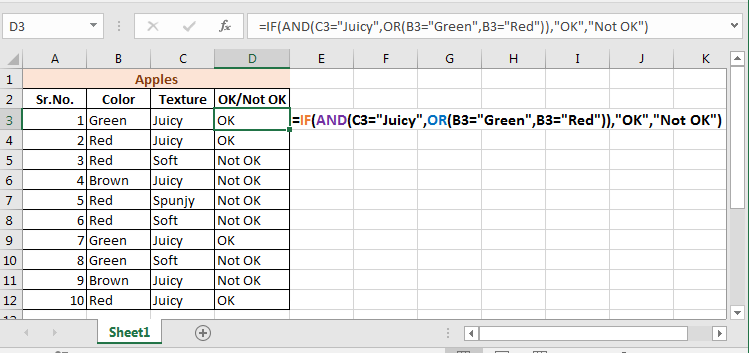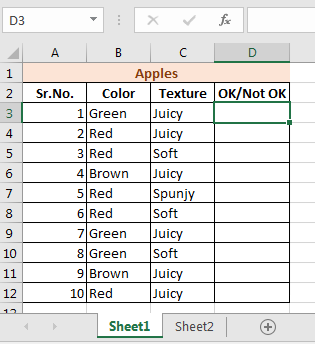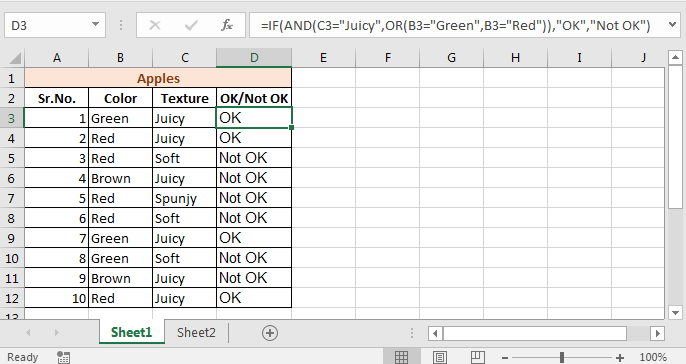# IF with AND and OR function in Excel

We learned about IF with AND Function in Excel and IF with OR Function in Excel previously. Now lets use AND function and OR function in one single formula.Scenario:
The fruit seller is again here. You would by an apple only if it is Juicy and Red or Green.
So here an apple must be Juicy but in color it can be Red or Green. We need an IF AND OR formula here.

#### IF This AND That OR That

Generic Formula

=IF(AND(condition1,OR(condition1, condition2,...)),value if true, value if false)

## Implementation of IF-AND-OR Formula

So to solve you problem of choosing apple you’ve drawn this table.Now to get right apples write this formula in cell D3 and drag it down.

=IF(AND(C3="Juicy",OR(B3="Green",B3="Red")),"OK","Not OK")This is the result.

## How It Works

It is simple.

OR(B3="Green",B3="Red") : This parts returns TRUE if B3 has Green or Red. Since it is green it returns TRUE.

AND(C3="Juicy",OR(B3="Green",B3="Red"): This part becomes AND(C3="Juicy",TRUE). AND returns TRUE only if C3 is Juicy and OR returns it TRUE. Since C3 is juicy and OR function has returned TRUE. this part will become AND(TRUE,TRUE). Which will eventually become TRUE.

IF(AND(C3="Juicy",OR(B3="Green",B3="Red")): This part becomes IF(TRUE,”OK”, “Not OK”). As we explained in IF Function article, it will return OK.

So yeah guys, this is the way you can pull conditions like if this and that or that type of conditions without using nested IFs function. Let me know if it was helpful in the comment section.

Related Articles:

IF function with Wildcards

IF with OR Function in Excel

IF with AND Function in Excel

Popular Articles:

50 Excel Shortcut to Increase Your Productivity

The VLOOKUP Function in Excel

COUNTIF in Excel 2016

How to Use SUMIF Function in Excel

Terms and Conditions of use

The applications/code on this site are distributed as is and without warranties or liability. In no event shall the owner of the copyrights, or the authors of the applications/code be liable for any loss of profit, any problems or any damage resulting from the use or evaluation of the applications/code.